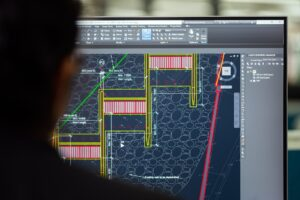# Principles of Earthwork Design: What Every Engineer Should Know

Every civil and environmental engineer should have a basic understanding of earthwork design principles. This knowledge is essential for designing and constructing roads, bridges, pipelines, and other infrastructure projects. In this article, we will discuss the most important concepts in earthwork design.

## Earthwork Design: What Is It?

In civil engineering, Earthwork Design is a process that is used to calculate the quantity and type of material that will be needed to construct a particular project. It also helps to determine the cost of the project and how long it will take to complete. In order to complete an accurate Earthwork Design, engineers need to know the dimensions of the project, as well as the type and density of the soil that will be used.

In order to analyse the soil, engineers use a variety of techniques, such as laboratory tests, field tests, and remote sensing. By using these techniques, they can determine the type and density of the soil, as well as its ability to support a particular weight. This information is essential for completing an accurate Earthwork Design.

Once the engineer has all of this information, they can begin to calculate the quantity and type of material that will be needed for the project. This calculation includes both the excavation and fill materials, as well as the necessary earthworks equipment. In addition, the engineer will also need to calculate the cost of the project and how long it will take to complete.

## Earthworks Design MethodsThere are a few different methods that can be used in Earthwork Design, but the most common is the Cut and Fill Method and the Three-Dimensional Method.

The Cut and Fill Method is the simplest way to calculate earthworks. This method involves dividing the project into a series of horizontal slices and then calculating the quantity of material that will be needed for each slice. The Three-Dimensional Method is more complicated, but it provides a more accurate estimate of earthworks. This method involves creating a three-dimensional model of the project and then calculating the quantity and type of material that will be needed.

### The Cut And Fill Method

In the Cut and Fill Method, the engineer first determines how much of the project will be cut (i.e. removed) and how much will be filled (i.e. added). He then calculates the volume of material that will be needed for each. This information is used to create a Material Takeoff, which lists the quantity and type of material required for the project.

The next step is to determine the cost of the material. This can be done by looking up the prices of each type of material on a price list, or by calculating the cost of excavation and transportation.

Finally, the engineer determines how long it will take to complete the project by calculating the number of manhours required. This information is used to create a Schedule of Activities, which shows when each phase of the project will be completed.

### The Three-Dimensional Method

The Three-Dimensional Method is more complicated than the Cut and Fill Method, but it provides a more accurate estimate of earthworks. The first step in this process is to create a Site Model. This is a CAD model of the project, which includes all of the dimensions and features of the site. The engineer then uses this model to calculate the quantity and type of material that will be needed.

The next step is to create a Material Database. This database includes information on the type and density of every type of soil in the area. By using this information, the engineer can accurately calculate the quantity and type of material that will be needed.

The final step is to create a Schedule of Activities. This schedule shows when each phase of the project will be completed, and how much material will be required for each.

## Which Method Should You Choose?

There is no one-size-fits-all answer to this question. The best method for a particular project will depend on the type of soil, the dimensions of the project, and the budget constraints. For example, the Cut and Fill Method may be preferable for a smaller project, while the Three-Dimensional Method may be needed for a large project with complex geometry. However, generally, most engineers prefer to use the Three-Dimensional Method whenever possible, because it provides the most accurate estimate of earthworks.

If you need to estimate the cost of earthworks for a project, there are two main methods: Cut and Fill or Three-Dimensional. The former is simpler but less accurate while the latter can be time-consuming and difficult, depending on your site’s geometry. I suggest using whichever method best fits your needs as an engineer – whether that means choosing the most accurate option or going for the quickest and most cost-effective one. Whichever you choose, make sure to research the prices of different types of materials and calculate the number of man-hours required for your project.